# how to do graph in linear inequalities and how to shade the solution region..... i cannot understand..?? plz help..

Graphing Linear Inequalities

This is a graph of a linear
inequality: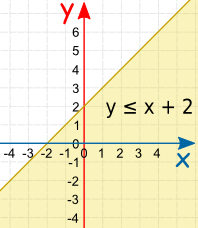The inequality y ≤ x + 2

You can see the y = x + 2 line, and the shaded area is where y is less than or equal to x + 2

Linear Inequality

A Linear Inequality is like a Linear Equation (such as y = 2x+1) ...

... but it will have an Inequality like <, >, ≤, or ≥ instead of an =.

How to Graph a Linear Inequality

First, graph the "equals" line, then shade in the correct area.

There are three steps:

• Rearrange the equation so "y" is on the left and everything else on the right.
• Plot the "y=" line (make it a solid line for y≤ or y≥, and a dashed line for y<or y>)
• Shade above the line for a "greater than" (y> or y≥)
or below the line for a "less than" (y< or y≤).

Let us try some examples:

Example: y≤2x-1

1. The inequality already has "y" on the left and everything else on the right, so no need to rearrange

2. Plot y=2x-1 (as a solid line because y≤ includes equal to)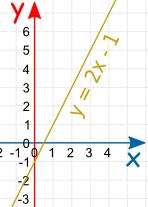3. Shade the area below (because y is less than or equal to)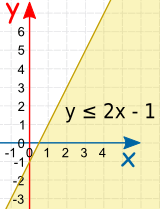Example: 2y − x ≤ 6

1. We will need to rearrange this one so "y" is on its own on the left:

Start with: 2y − x ≤ 6 Add x to both sides: 2y ≤ x + 6 Divide all by 2: y ≤ x/2 + 3

2. Now plot y = x/2 + 3 (as a solid line because y≤ includes equal to)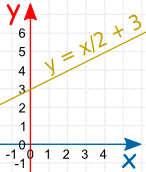3. Shade the area below (because y is less than or equal to)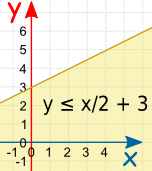Example: y/2 + 2 > x

1. We will need to rearrange this one so "y" is on its own on the left:

Start with: y/2 + 2 > x Subtract 2 from both sides: y/2 > x − 2 Multiply all by 2: y > 2x − 4

2. Now plot y = 2x − 4 (as a dashed line because y> does not include equals to)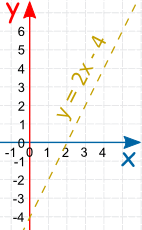3. Shade the area above (because y is greater than)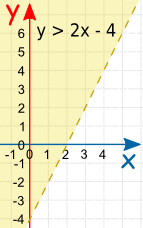The dashed line shows that the inequality does not include the line y=2x-4.

Two Special Cases

You could also have a horizontal or vertical line: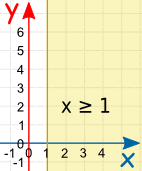This shows where y is less than 4
(from, but not including, the line y=4 on down)
Notice that we have a dashed line to show that it does not include where y=4 This one doesn't even have y in it!
It has the line x=1, and is shaded for all values of x greater than (or equal to) 1
• 1
What are you looking for?